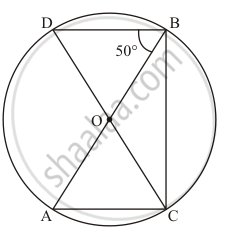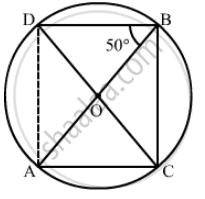Advertisement Remove all ads

# In the Given Figure, Ab and Cd Are Diameters of a Circle with Centre O. If ∠Obd = 50°, Find ∠Aoc. - Mathematics

Short Note

In the given figure, AB and CD are diameters of a circle with centre O. If ∠OBD = 50°, find ∠AOC.Advertisement Remove all ads

#### Solution

It is given that, AB and CD are diameter with center O and angleOBD = 50°We have to find angle AOC

Construction: Join the point A and D to form line AD

Clearly arc AD subtendsangle ABD = 50°  at B and angleAOD  at the centre.

Therefore,

$\angle AOD = 2\angle ABD = 100°$         …… (1)

Since CD is a straight line then

$\angle DOA + \angle AOC = 180° \left( \text{ Linear pair } \right)$
angle AOC = 180° - 100°
= 80°
Hence `angle AOC = 80°

Is there an error in this question or solution?
Advertisement Remove all ads

#### APPEARS IN

RD Sharma Mathematics for Class 9
Chapter 15 Circles
Exercise 15.5 | Q 7 | Page 101
Advertisement Remove all ads
Advertisement Remove all ads
Share
Notifications

View all notifications

Forgot password?
Course